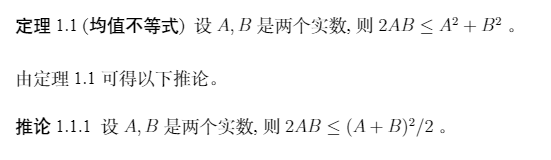# # 📒 本科生毕业设计：毕业论文

🚀 更新提示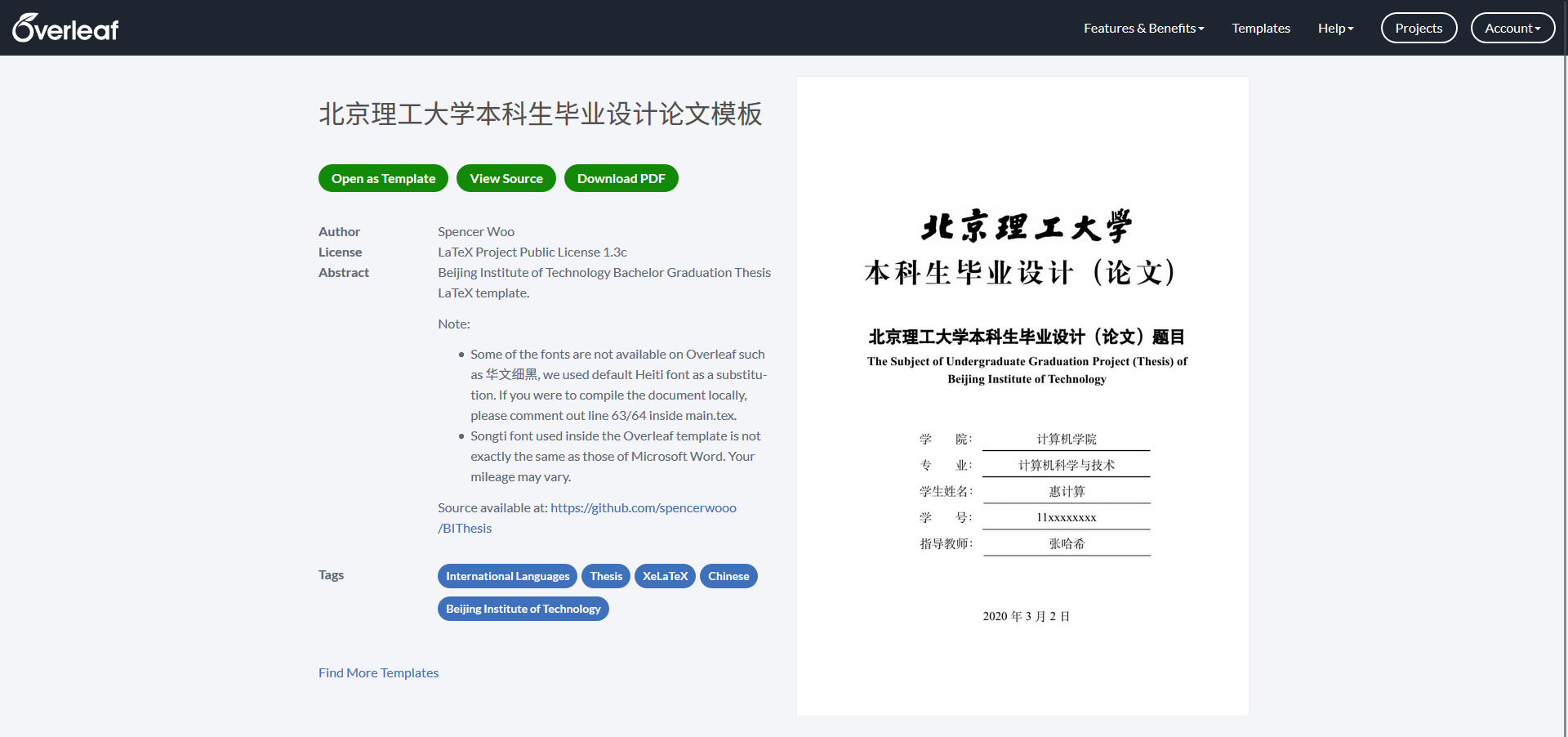Overleaf 版本的毕业论文模板中由于没有微软版权字体「华文细黑」，导致封面的毕业论文中文大标题无法用 Word 模板中规定的字体渲染，使得最终呈现样式与要求有些出入，如果希望保证 LaTeX 模板输出和学校模板一致，那么还是推荐在本地进行撰写和编译。

## # 熟悉项目

.
├── chapters
│    ├── 0_abstract.tex
│    └── 1_chapter1.tex
├── images
│    ├── bit_logo.png
└── misc
│    ├── 0_cover.tex
│    ├── 1_originality.tex
│    ├── 2_toc.tex
│    ├── 3_conclusion.tex
│    ├── 4_reference.tex
│    ├── 5_appendix.tex
│    ├── 6_acknowledgements.tex
│    └── ref.bib
├── main.tex
└── main.pdf


• main.tex：毕业论文模板的主文件
• ./chapters 文件夹：包含有整个毕业论文的「摘要」和正文的全部「章节」
• 0_abstract.tex：毕业论文的「摘要」（中文摘要与英文摘要）
• 1_chapter1.tex：毕业论文正文「第一章」（示例章节）
• ……（你可以继续添加第二章 2_chapter2.tex、第三章 3_chapter3.tex……，并在主文件 main.tex 中引用（详见下文）
• ./misc 文件夹：包含有毕业论文模板中的封面、后置章节与参考文献
• 0_cover.tex：毕业论文的「封面」，一般情况无需更改
• 1_originality.tex：毕业论文的「原创性声明」，一般情况无需更改（签字和日期后期手动添加）
• 1_originality.pdf：毕业论文的「原创性声明」，是由原 Word 模板导出的一页 PDF 文档，插入到毕业论文中的（如果上一行中的 tex 版本格式无法满足你的需要，可以用 Word 将这一单页导出为 PDF 插入。详见源 LaTeX 代码）
• 2_toc.tex：毕业论文的「目录」，一般情况无需更改（由 LaTeX 自动生成）
• 3_conclusion.tex：毕业论文的「结论」，按照一般章节文件对待
• 4_reference.tex：毕业论文的「参考文献」，一般情况无需更改（由 LaTeX 根据你文档中的 \cite{} 自动生成）
• 5_appendix.tex：毕业论文的「附录」，按照一般章节文件对待
• 6_acknowledgements.tex：毕业论文的「致谢」，按照一般章节文件对待
• ref.bib：参考文献 BibTeX 数据库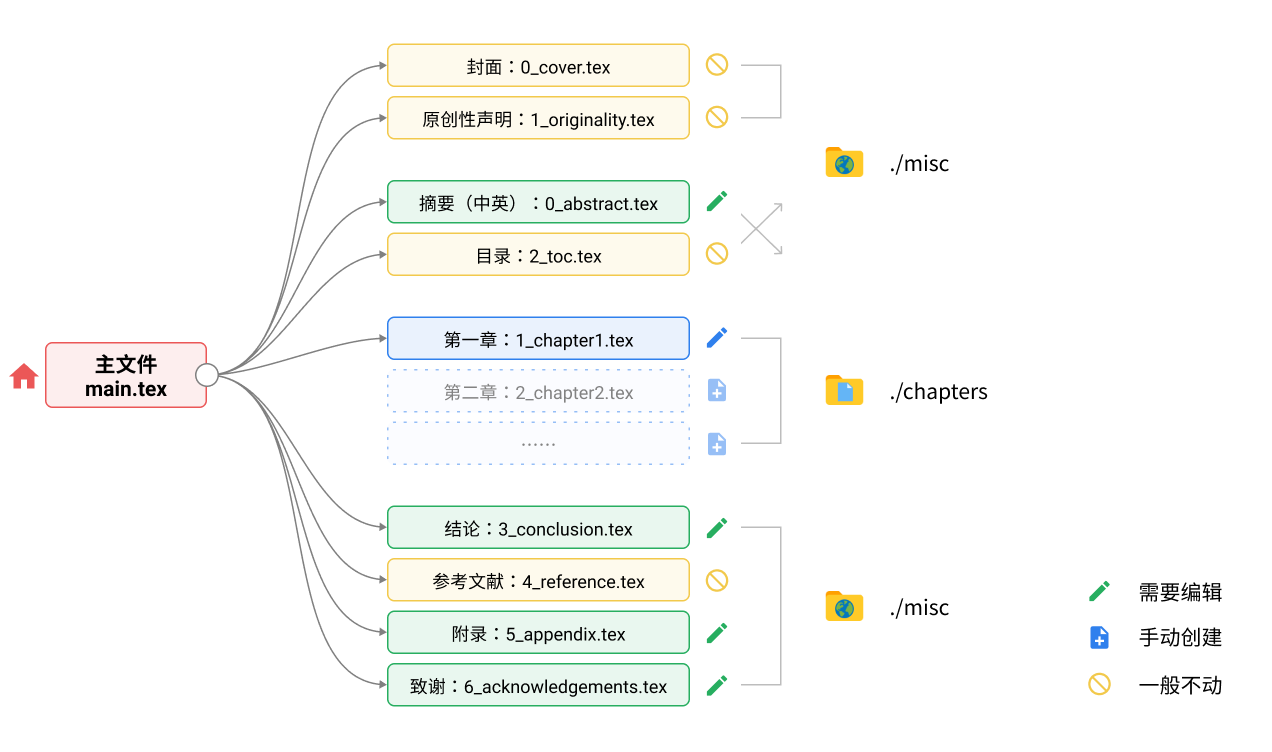## # 你的内容从哪里开始？

### # 开始

• 你可以通过控制 \thesisTitle 这一变量来控制整个论文的「中文标题」
• 你可以通过控制 \thesisTitleEN 这一变量来控制整个项目的「英文标题」

• \deptName：你所在学院
• \majorName：你所就读的专业
• \yourName：你的姓名
• \yourStudentID：你的学号
• \mentorName：你的指导教师

• \externalMentorName：你的校外指导教师

### # 正文

% 第一章
\input{chapters/1_chapter1.tex}
% 在这里添加第二章、第三章……TeX 文件的引用
\input{chapters/2_chapter2.tex}
\input{chapters/3_chapter3.tex}


### # 后续模块

• Conclusion 结论：misc/3_conclusion.tex
• Reference 参考文献：misc/4_reference.tex
• Appendix 附录：misc/5_appendix.tex
• Acknowledgements 致谢：misc/6_acknowledgements.tex

## # 参考文献管理

BibTeX 是一种表示、存储与引用参考文献的语法，谷歌学术中搜索文章直接复制得到。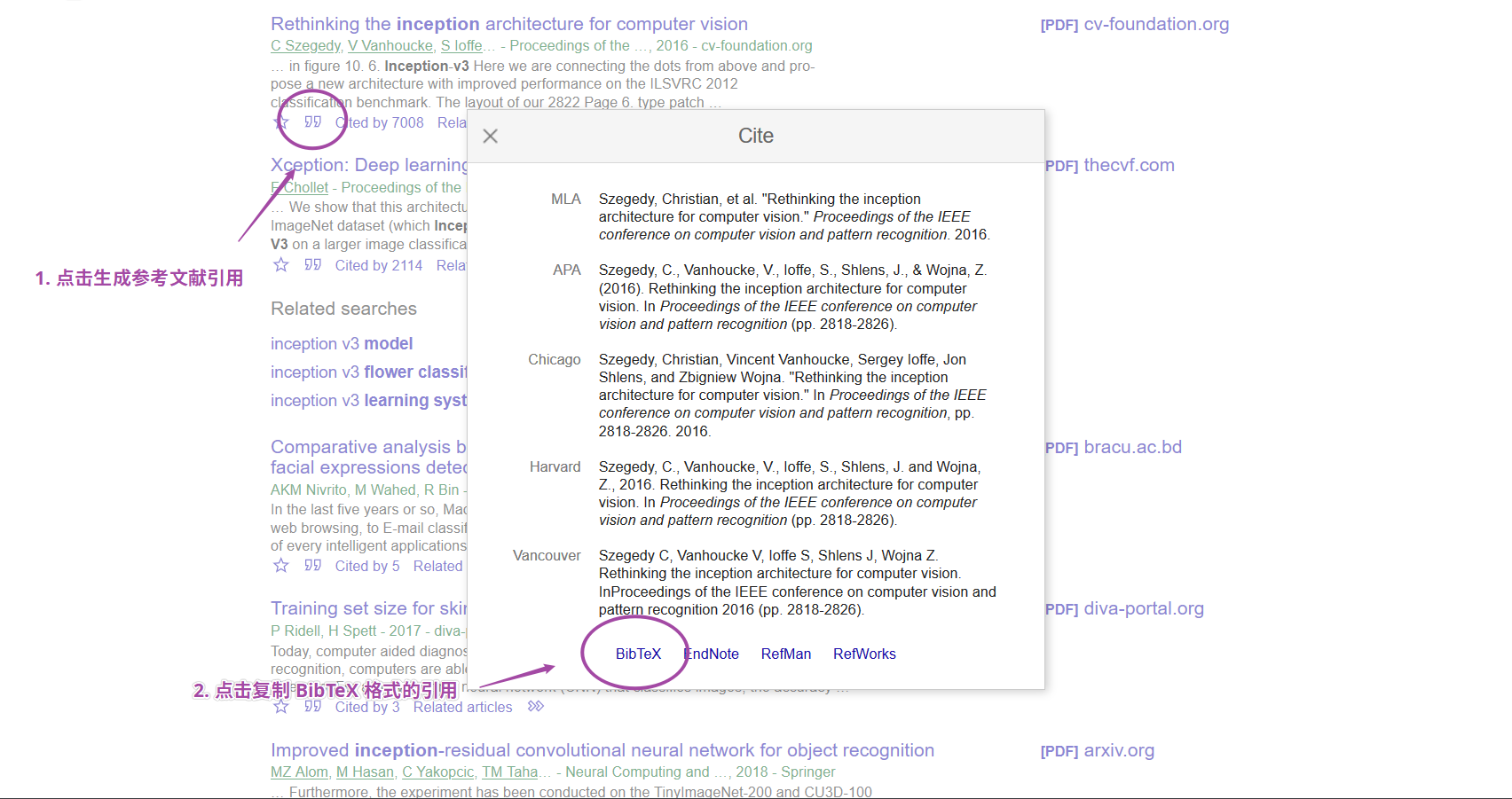@inproceedings{szegedy2016rethinking,
title={Rethinking the inception architecture for computer vision},
author={Szegedy, Christian and Vanhoucke, Vincent and Ioffe, Sergey and Shlens, Jon and Wojna, Zbigniew},
booktitle={Proceedings of the IEEE conference on computer vision and pattern recognition},
pages={2818--2826},
year={2016}
}


正文，正文正文 \cite{szegedy2016rethinking} 正文正文……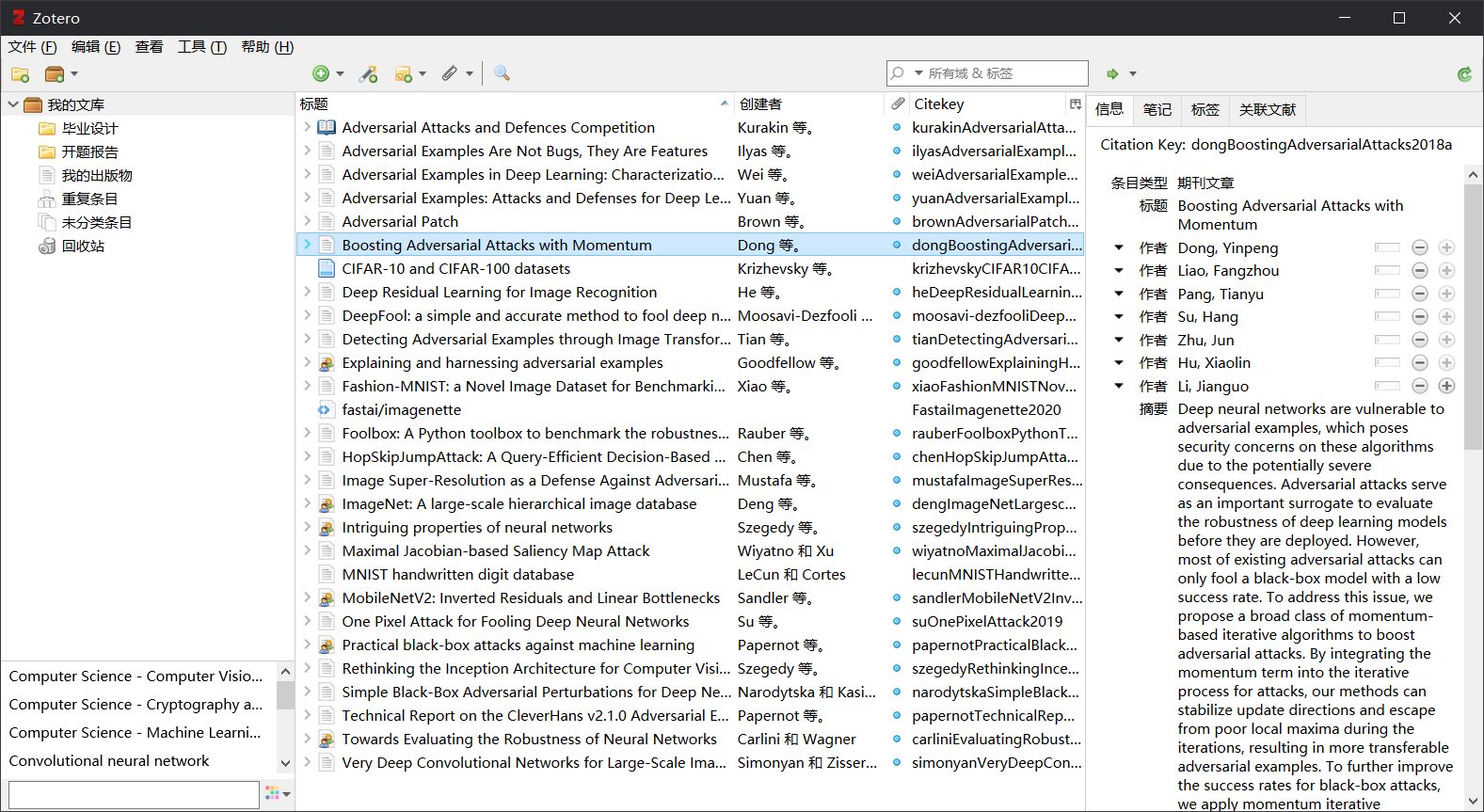## # 图片素材

\begin{figure}[htbp]
\vspace{13pt} % 调整图片与上文的垂直距离
\centering
\includegraphics[width=0.8\textwidth]{images/bit_logo.png}
\caption{标题序号}\label{标题序号} % label 用来在文中索引
\end{figure}


• 我们首先将图片放置在了一个 \begin{figure} ... \end{figure} 的环境中，其中 [htbp] 是用于定位图片。
• 之后，在环境中，我们首先使用 \centering 保证图片水平居中
• 之后用 \includegraphics[图片大小]{图片路径} 的格式引用了图片本身（图片大小的语法width=0.8\textwidth 表示图片宽度是整个页宽的 0.8 倍）
• 最后我们定义了图片的说明文字 \caption{图片说明} 和图片的标签编号 \label{图片编号}，前者显示在图片下方起到说明注释的作用，后者让我们可以用 \ref{图片编号} 的语法来在正文中引用图片

\begin{figure}[htbp]
\vspace{13pt} % 调整图片与上文的垂直距离
\centering
\caption{标题序号}\label{标题序号} % label 用来在文中索引
\end{figure}


• \includesvg 宏内引用 SVG 图片的文件名称时，不需要携带 .svg 扩展名，而是直接引用文件名称；
• 请务必使用 \adjustbox 宏来对 SVG 图片的大小进行调整，而不要使用 \includesvg[width=0.8\textwidth] 这样的方式对图片大小进行调整，因为后者无法很好地处理 SVG 图片中文字的缩放。

## # 公式插入

LaTeX 的行内数学符号和公式等，使用 $$...$$ 的语法进行定义。比如类似如下的正文：

The well known Pythagorean theorem $$x^2 + y^2 = z^2$$ was
proved to be invalid for other exponents.
Meaning the next equation has no integer solutions:

$x^n + y^n = z^n$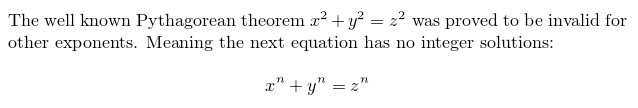In physics, the mass-energy equivalence is stated
by the equation $E=mc^2$, discovered in 1905 by Albert Einstein.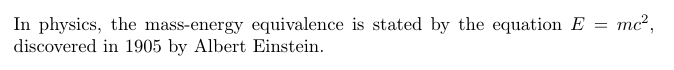\begin{equation}
LRI=1\ ∕\ \sqrt{1+{\left(\frac{{\mu }_{R}}{{\mu }_{s}}\right)}^{2}{\left(\frac{{\delta }_{R}}{{\delta }_{s}}\right)}^{2}}
\end{equation}## # 其他

### # 代码高亮

• 在文档开题引入宏包：

\usepackage{minted}

• 渲染代码块：

\begin{minted}{python}
import numpy as np

def incmatrix(genl1,genl2):
m = len(genl1)
n = len(genl2)
M = None #to become the incidence matrix
VT = np.zeros((n*m,1), int)  #dummy variable

#compute the bitwise xor matrix
M1 = bitxormatrix(genl1)
M2 = np.triu(bitxormatrix(genl2),1)
\end{minted}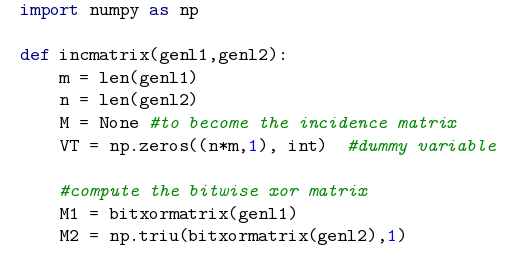### # 算法模块

• 在文档开头引入宏包：

\usepackage[ruled,vlined]{algorithm2e}

• 渲染伪代码模块：

\begin{algorithm}[H]
\SetAlgoLined
\KwResult{Write here the result }
initialization\;
\While{While condition}{
instructions\;
\eIf{condition}{
instructions1\;
instructions2\;
}{
instructions3\;
}
}
\caption{How to write algorithms}
\end{algorithm}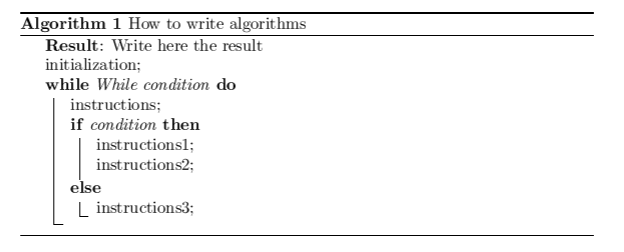### # 定理插入

• 在文档开头定义环境：

\newtheorem{theorem}{\hspace{2em}定理}[chapter]
\newtheorem{corollary}{\hspace{2em}推论}[theorem]

% 上述每行命令第一个大括号中为环境名称，
% 第二个大括号中为该环境渲染的模块标题，
% 方括号中选项用来设置编号逻辑，如 [chapter] 表示第 n 章节中定理编号依次为 n.1, n.2, …… 。

• 渲染定理及推论模块：

\begin{theorem}[均值不等式]
\label{thm-1}
设 $A,B$ 是两个实数, 则 $2AB\leq A^2+B^2$。
\end{theorem}

由定理 \ref{thm-1} 可得以下推论。

\begin{corollary}
设 $A,B$ 是两个实数, 则 $2AB\leq (A+B)^2/2$。
\end{corollary}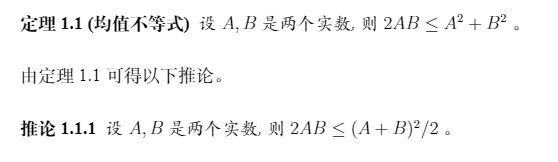• 类似的，你还可以定义引理、定义、命题等环境：

\newtheorem{lemma}{\hspace{2em}引理}[chapter]
\newtheorem{definition}{\hspace{2em}定义}[chapter]
\newtheorem{proposition}{\hspace{2em}命题}[chapter]


• 在文档开头引入宏包，并指定定理环境的字体格式：

\usepackage{ntheorem}
\theoremstyle{plain}